# Linux Shell 运算符

Shell 和其他编程语言一样，支持多种运算符，包括：

• 算数运算符
• 关系运算符
• 布尔运算符
• 逻辑运算符
• 字符串运算符
• 文件测试运算符

expr 是一款表达式计算工具，使用它能完成表达式的求值操作。

#!/bin/bash

val=expr 2 + 2
echo "两数之和为 : $val" 执行脚本，输出结果如下所示： 两数之和为 : 4 两点注意： • 表达式和运算符之间要有空格，例如 2+2 是不对的，必须写成 2 + 2，这与我们熟悉的大多数编程语言不一样。 • 完整的表达式要被   包含，注意这个字符不是常用的单引号，在 Esc 键下边。 一、算数运算符 下表列出了常用的算术运算符，假定变量 a 为 10，变量 b 为 20：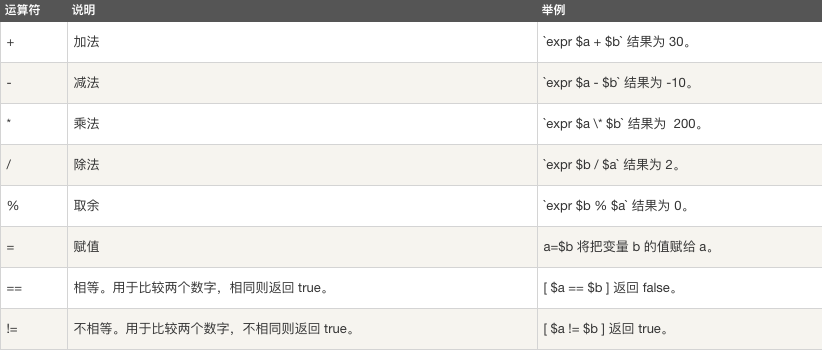注意：条件表达式要放在方括号之间，并且要有空格，例如: [$a==$b] 是错误的，必须写成 [$a == $b ] 实例 a=10 b=20 val=expr$a + $b echo "a + b :$val"

val=expr $a -$b
echo "a - b : $val" val=expr$a \* $b echo "a * b :$val"

val=expr $b /$a
echo "b / a : $val" val=expr$b % $a echo "b % a :$val"

if [ $a ==$b ]
then
echo "a 等于 b"
fi
if [ $a !=$b ]
then
echo "a 不等于 b"
fi

a + b : 30
a - b : -10
a * b : 200
b / a : 2
b % a : 0
a 不等于 b 

• 乘号(*)前边必须加反斜杠(\)才能实现乘法运算；
• if...then...fi 是条件语句，后续将会讲解。
• 在 MAC 中 shell 的 expr 语法是：$((表达式))，此处表达式中的 "*" 不需要转义符号 "\" 。 二、关系运算符 关系运算符只支持数字，不支持字符串，除非字符串的值是数字。 下表列出了常用的关系运算符，假定变量 a 为 10，变量 b 为 20：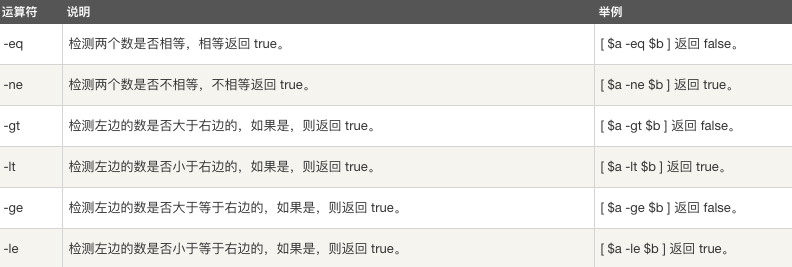### 实例 a=10 b=20 if [$a -eq $b ] then echo "$a -eq $b : a 等于 b" else echo "$a -eq $b: a 不等于 b" fi if [$a -ne $b ] then echo "$a -ne $b: a 不等于 b" else echo "$a -ne $b : a 等于 b" fi if [$a -gt $b ] then echo "$a -gt $b: a 大于 b" else echo "$a -gt $b: a 不大于 b" fi if [$a -lt $b ] then echo "$a -lt $b: a 小于 b" else echo "$a -lt $b: a 不小于 b" fi if [$a -ge $b ] then echo "$a -ge $b: a 大于或等于 b" else echo "$a -ge $b: a 小于 b" fi if [$a -le $b ] then echo "$a -le $b: a 小于或等于 b" else echo "$a -le $b: a 大于 b" fi 执行脚本，输出结果如下所示： 10 -eq 20: a 不等于 b 10 -ne 20: a 不等于 b 10 -gt 20: a 不大于 b 10 -lt 20: a 小于 b 10 -ge 20: a 小于 b 10 -le 20: a 小于或等于 b 三、布尔运算符 下表列出了常用的布尔运算符，假定变量 a 为 10，变量 b 为 20：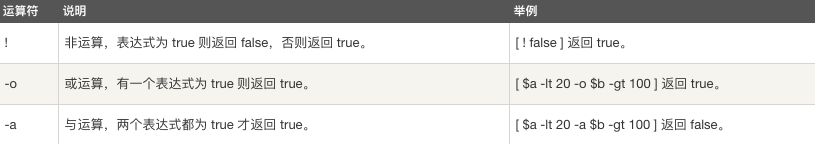实例 #!/bin/bash # author:菜鸟教程 # url:www.runoob.com a=10 b=20 if [$a != $b ] then echo "$a != $b : a 不等于 b" else echo "$a != $b: a 等于 b" fi if [$a -lt 100 -a $b -gt 15 ] then echo "$a 小于 100 且 $b 大于 15 : 返回 true" else echo "$a 小于 100 且 $b 大于 15 : 返回 false" fi if [$a -lt 100 -o $b -gt 100 ] then echo "$a 小于 100 或 $b 大于 100 : 返回 true" else echo "$a 小于 100 或 $b 大于 100 : 返回 false" fi if [$a -lt 5 -o $b -gt 100 ] then echo "$a 小于 5 或 $b 大于 100 : 返回 true" else echo "$a 小于 5 或 \$b 大于 100 : 返回 false"
fi

10 != 20 : a 不等于 b
10 小于 100 且 20 大于 15 : 返回 true
10 小于 100 或 20 大于 100 : 返回 true
10 小于 5 或 20 大于 100 : 返回 false`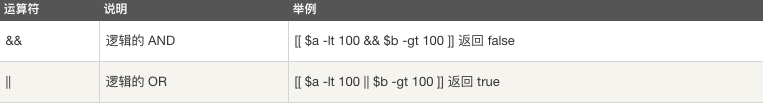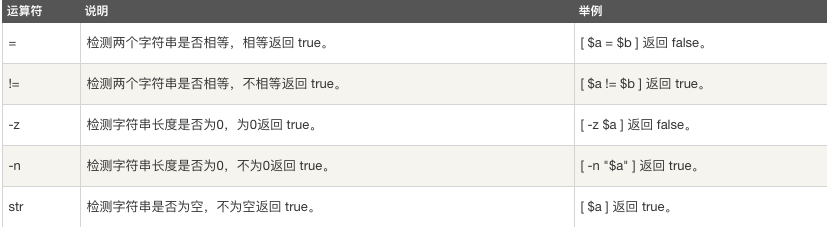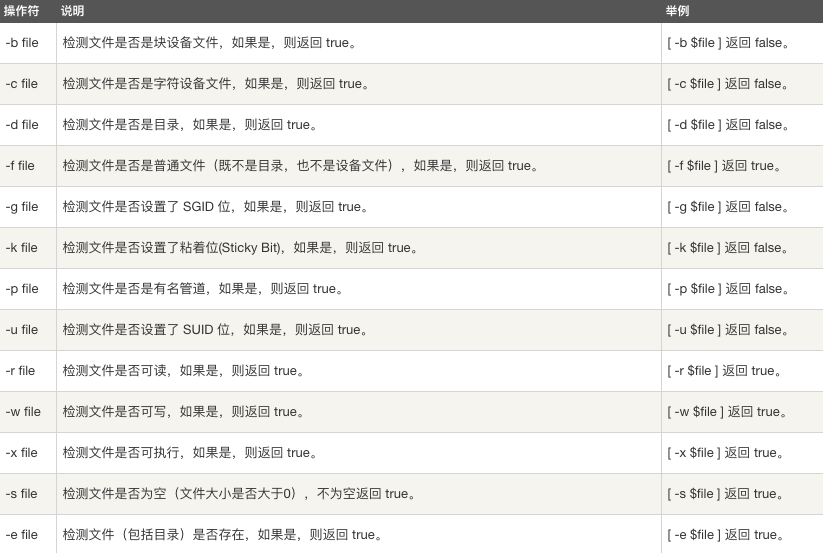posted on 2018-10-18 17:26  海燕。  阅读(2292)  评论(0编辑  收藏  举报